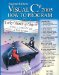# foreach Statement

In previous examples, we demonstrated how to use counter-controlled for statements to iterate through the elements in an array. In this section, we introduce the foreach statement, which iterates through the elements of an entire array or collection. This section discusses how to use the foreach statement to loop through an array. We show how to use the foreach statement with collections in Chapter 27, Collections. The syntax of a foreach statement is:

```foreach ( type identifier in arrayName )
statement
```

where type and identifier are the type and name (e.g., int number) of the iteration variable, and arrayName is the array through which to iterate. The type of the iteration variable must match the type of the elements in the array. As the next example illustrates, the iteration variable represents successive values in the array on successive iterations of the foreach statement.

Figure 8.12 uses the foreach statement (lines 1314) to calculate the sum of the integers in an array of student grades. The type specified is int, because array contains int valuestherefore, the loop will select one int value from the array during each iteration. The foreach statement iterates through successive values in the array one-by-one. The foreach header can be read concisely as "for each iteration, assign the next element of array to int variable number, then execute the following statement." Thus, for each iteration, identifier number represents the next int value in the array. Lines 1314 are equivalent to the following counter-controlled repetition used in lines 1314 of Fig. 8.5 to total the integers in array:

```for ( int counter = 0; counter < array.Length; counter++ )
total += array[ counter ];
```

Figure 8.12. Using the foreach statement to total integers in an array.

``` 1 // Fig. 8.12: ForEachTest.cs
2 // Using foreach statement to total integers in an array.
3 using System;
4
5 public class ForEachTest
6 {
7 public static void Main( string[] args )
8 {
9 int[] array = { 87, 68, 94, 100, 83, 78, 85, 91, 76, 87 };
10 int total = 0;
11
12 // add each element's value to total
13 foreach ( int number in array )
14  total += number;
15
16 Console.WriteLine( "Total of array elements: {0}", total );
17 } // end Main
18 } // end class ForEachTest
```

 ``` Total of array elements: 849 ```

The foreach statement simplifies the code for iterating through an array. Note, however, that the foreach statement can be used only to access array elementsit cannot be used to modify elements. Any attempt to change the value of the iteration variable in the body of a foreach statement will cause a compilation error. If your application needs to modify elements, use the for statement.

The foreach statement can be used in place of the for statement whenever code looping through an array does not require access to the counter indicating the index of the current array element. For example, totaling the integers in an array requires access only to the element valuesthe index of each element is irrelevant. However, if an application must use a counter for some reason other than simply to loop through an array (e.g., to print an index number next to each array element value, as in the examples earlier in this chapter), use the for statement.

### Passing Arrays and Array Elements to MethodsVisual C# 2005 How to Program (2nd Edition)
ISBN: 0131525239
EAN: 2147483647
Year: 2004
Pages: 600